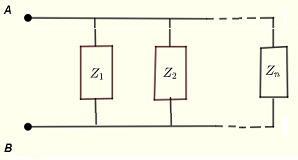# Parallel Impedance Calculator

A calculator of the equivalent impedance to a group of impedances in parallel is presented.

## Impedances in Parallel

The impedance $Z$ that is equivalent to the impedances $Z_1$, $Z_12$ .... $Z_n$ grouped in parallel, as shown below, is given by$\dfrac{1}{Z} = \dfrac{1}{Z_1} + \dfrac{1}{Z_2} + ... + \dfrac{1}{Z_n}$
or
$Z = \dfrac{1}{ \dfrac{1}{Z_1} + \dfrac{1}{Z_2} + ... + \dfrac{1}{Z_n} }$

## Example of Parallel Impedances

There are 4 parallel impedances in the circuit below,In complex form, the impedances $Z_1$, $Z_12$, $Z_3$ and $Z_4$ are written as
$Z_1 = R_1 + 0 \; j$
$Z_2 = 0 + \omega L_1 \; j$
$Z_3 = R_2 - \dfrac{1}{ \omega \; C_1 } \; j$
$Z_4 = R_3 + \left( \omega L_2 - \dfrac{1}{ \omega \; C_1 } \right) \; j$
where $j$ is the imaginary unit.
The equivalent impedance $Z$ seen between points A and B is given by
$Z = \dfrac{1}{ \dfrac{1}{Z_1} + \dfrac{1}{Z_2} + \dfrac{1}{Z_3} + \dfrac{1}{Z_3} }$
The numerical values of the resistances, capacitances and inductances included in the circuit and the frequency of the input voltage are needed to express the impedances numerically and use them in the calculator below.
More AC circuits calculators and solvers are included.

## How to Use the Calculator

Enter the number $n$ of impedances in parallel as a whole number and press "Enter".
Then enter the values of the impedances as complex numbers of the form $a + b j$, where the real part $a$ is on the left column of the table and the imaginary part $b$ is on the right column of the table and press "Update/Calculate".
The outputs include all the impedances entered which may be checked and modified if necessary as well as the equivalent impedance $Z$ in complex standard and polar forms.
Note that each impedance must have a real part and an imaginary part and if one of the two parts is equal to 0 you must enter the number 0 for that part.
Number of Impedances: $n =$

Change values of impedances (if needed) and